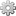# Formula MacroInserts a mathematical formula
 Type JAR Category Developed by Unknown Active Installs 0 Rating 0 Votes License GNU Lesser General Public License 2.1
 Compatibility Starting with XWiki 14.7RC1, the formula Macro has been extracted out as a Contrib Extension.

Installable with the Extension Manager

# Description

The formula needs is written in TeX markup and rendered as an image on the server.

# Usage

Examples of formula syntax can be seen on the Wikipedia Markup page.

## Parameters

• imageType: The image format; possible values: png (default), gif, jpg
• fontSize: "Fuzzy" sizes, corresponding to the LaTeX font sizes:
• tiny (LaTeX:\tiny),
• very_small (LaTeX:\scriptsize),
• smaller (LaTeX:\footnotesize),
• small (LaTeX:\small),
• normal (default) (LaTeX:\normalsize),
• large (LaTeX:\large),
• larger (LaTeX:\Large),
• very_large (LaTeX:\LARGE),
• huge (LaTeX:\huge),
• extremely_huge (LaTeX:\Huge).

## Inserting a formula in the wiki editor

{{formula fontSize="small"}}\sum_{n=1}^\infty\frac{1}{n^2} = \frac{\pi^2}{6}{{/formula}}

## Inserting a formula in the WYSIWYG editor

Place the TeX markup corresponding to the formula in the content field of the macro insertion dialog:## Inline vs. block formula

The formula macro can be used inline, inside a paragraph, or as a standalone block. Some differences exist between the two results: just like in LaTeX, inline formulas take up less vertical space than block formulas. For example, the code

Inline, a formula looks like this: {{formula}}\sum_{n=1}^\infty\frac{1}{n^2}=\frac{\pi^2}{6}{{/formula}}, while standalone it looks like this:

{{formula}}\sum_{n=1}^\infty\frac{1}{n^2}=\frac{\pi^2}{6}{{/formula}}

generates# Technical information

The macro uses third-party tools for generating the image corresponding to a mathematical formula. Currently, four alternatives exist:

• one based on native system calls to latex, and additional conversion tools depending on the image type. It gives the best results from a graphical point of view, but requires the presence of external programs, and involves a slight overhead by starting new processes and working with the disk.
• When rendering formulas in PNG, JPEG or GIF, the utilities dvips and convert (ImageMagick) should be installed
• Since 13.10-rc-1 When rendering formulas in SVG, the utility dvisvgm should be installed
• one based on MathTran as a remote service through HTTP requests. The graphic results are as good as the ones obtained by the native method. Additionally, the image contains some useful metadata: the actual formula that generated the image, the position of the baseline for proper alignment, and any errors that may have occurred while processing the request. The disadvantage of this approach is that it relies heavily on a remote server.
• the third implementation uses SnuggleTeX and JEuclid to transform LaTeX into MathML, and then render it into images. The results are not as eye-pleasing as those obtained from LaTeX, but it is a 100% Java, self-contained solution, with no external dependencies.
• a fourth one was introduced in XWiki 2.1, based on the public Google Chart APIs as a remote service through HTTP requests. The quality of the results is between those obtained from the native TeX system and those from SnuggleTeX. Unlike MathTran, it doesn't use a native TeX system, thus there are a few disadvantages other than the quality:
• only block-level equations are produced
• there's no support (yet?) for different font sizes and image formats
• the results are not 100% in accordance with the TeX syntax (for example it does not understand root, the content between left and right is lowered)

The method used by default is the one based on native system calls. If the required tools are not available on the system, the SnuggleTeX implementation is used instead.

## Configuration

The macro provides a couple of configuration keys to be defined in xwiki.properties.

• macro.formula.renderer: allows to define the default renderer to be used when rendering formulas. The possible values are native, mathtran, snuggletex and googlecharts.
• macro.formula.defaultType: the default image type to be used when rendering a formula. The possible values are PNG, GIF, JPEG or SVG. If the property is not defined, PNG will be used.
• 14.3+ macro.formula.defaultFontSize: the default font size to be used when rendering a formula. The possible values are the same as the fontSize parameter, but captialized (eg NORMAL, HUGE, ...)

# Troubleshooting

Depending on the XWiki installation, some characters (like \phi, \omega, etc.) may not be displayed. In this case, make sure GNU FreeFont (gnu-free-serif-fonts) is installed.

# Acknowledgments

This feature is based on the Tex plugin developed by Guillaume Legris.

# Prerequisites & Installation Instructions

We recommend using the Extension Manager to install this extension (Make sure that the text "Installable with the Extension Manager" is displayed at the top right location on this page to know if this extension can be installed with the Extension Manager).

You can also use the manual method which involves dropping the JAR file and all its dependencies into the WEB-INF/lib folder and restarting XWiki.

14.7+

You need to manually modify the WEB-INF/cache/infinispan/config.xml file to add the following:

<!-- Formula Renderer cache -->
<local-cache-configuration name="xwiki.plugin.formula">
<persistence>
<!-- Let XWiki cache module set the proper path -->
<file-store segmented="false" purge="true" path="" />
</persistence>
</local-cache-configuration>

# Dependencies

Dependencies for this extension (org.xwiki.contrib.formula:macro-formula-macro 14.6):

Tags: math latex